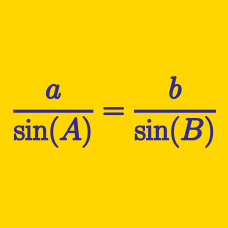Geometry

# Solving Triangles: Level 4 Challenges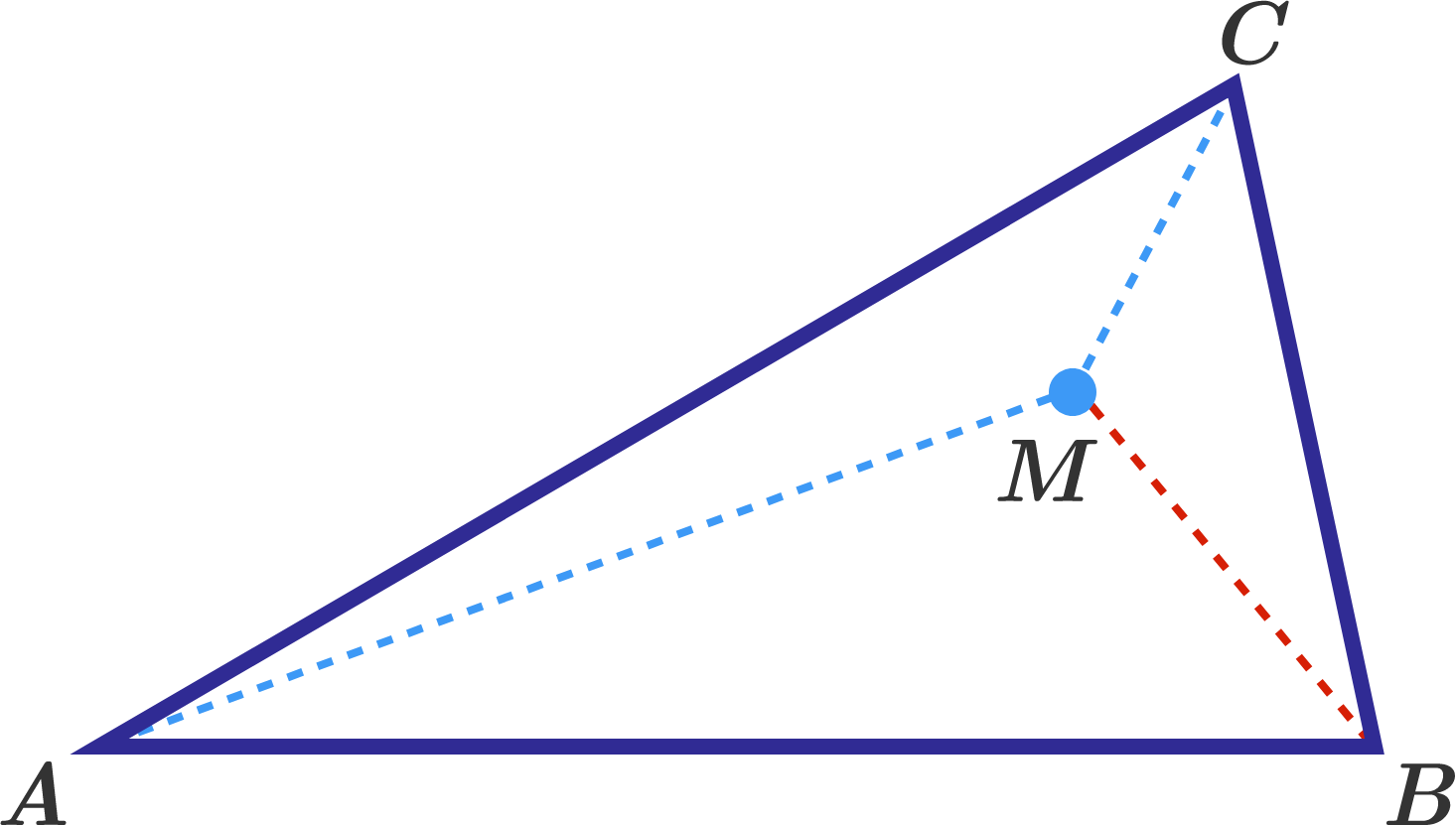In a triangle $$ABC$$, the measures of the angles are $$\angle A=30^\circ,$$ $$\angle B=80^\circ.$$ Point $$M$$ lies inside the triangle, such that $$\angle MAC = 10^\circ,$$ $$\angle MCA = 30^\circ.$$ What is the measure (in degrees) of $$\angle BMC?$$

Triangle $$ABC$$ is isosceles with $$AC = BC$$ and $$\angle ACB = 106^\circ$$ Point $$M$$ is inside the triangle so that $$\angle MAC = 7^\circ$$ and $$\angle MCA = 23^\circ$$. Find the measure of $$\angle CMB$$ in degrees.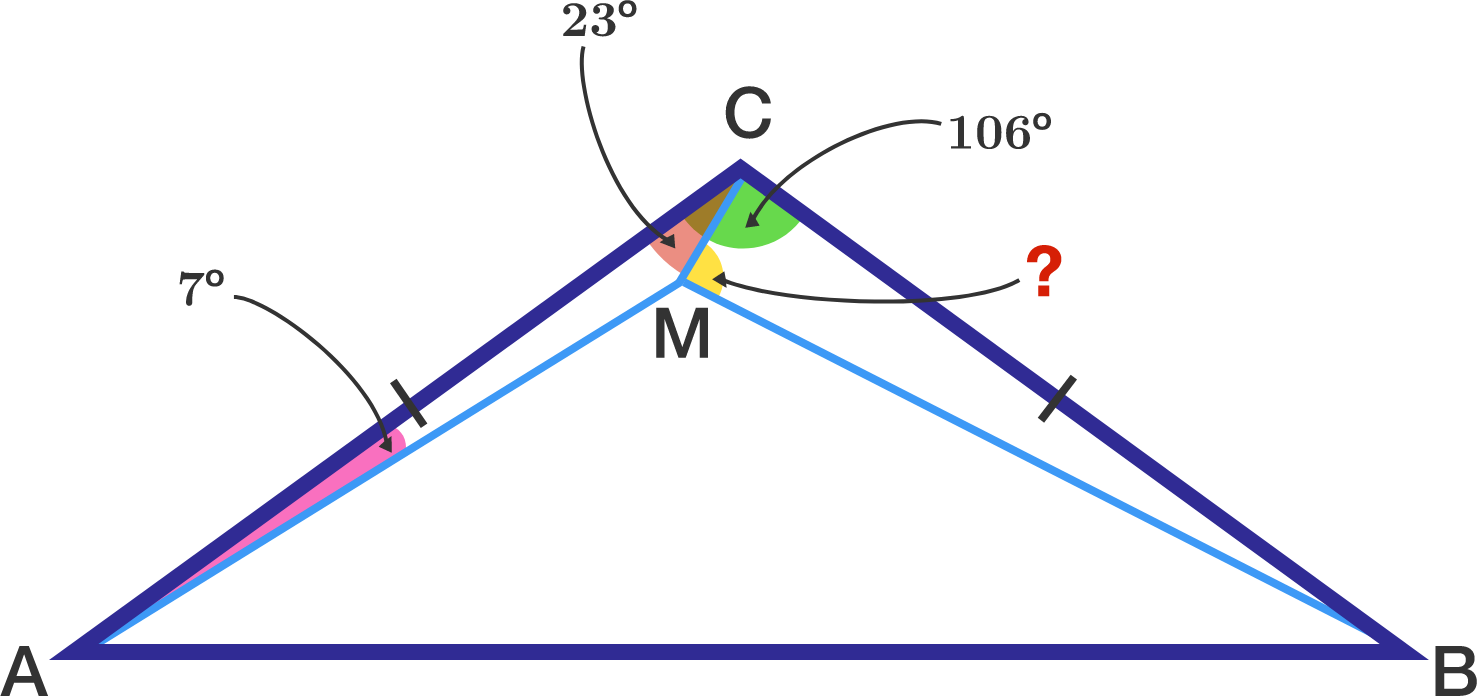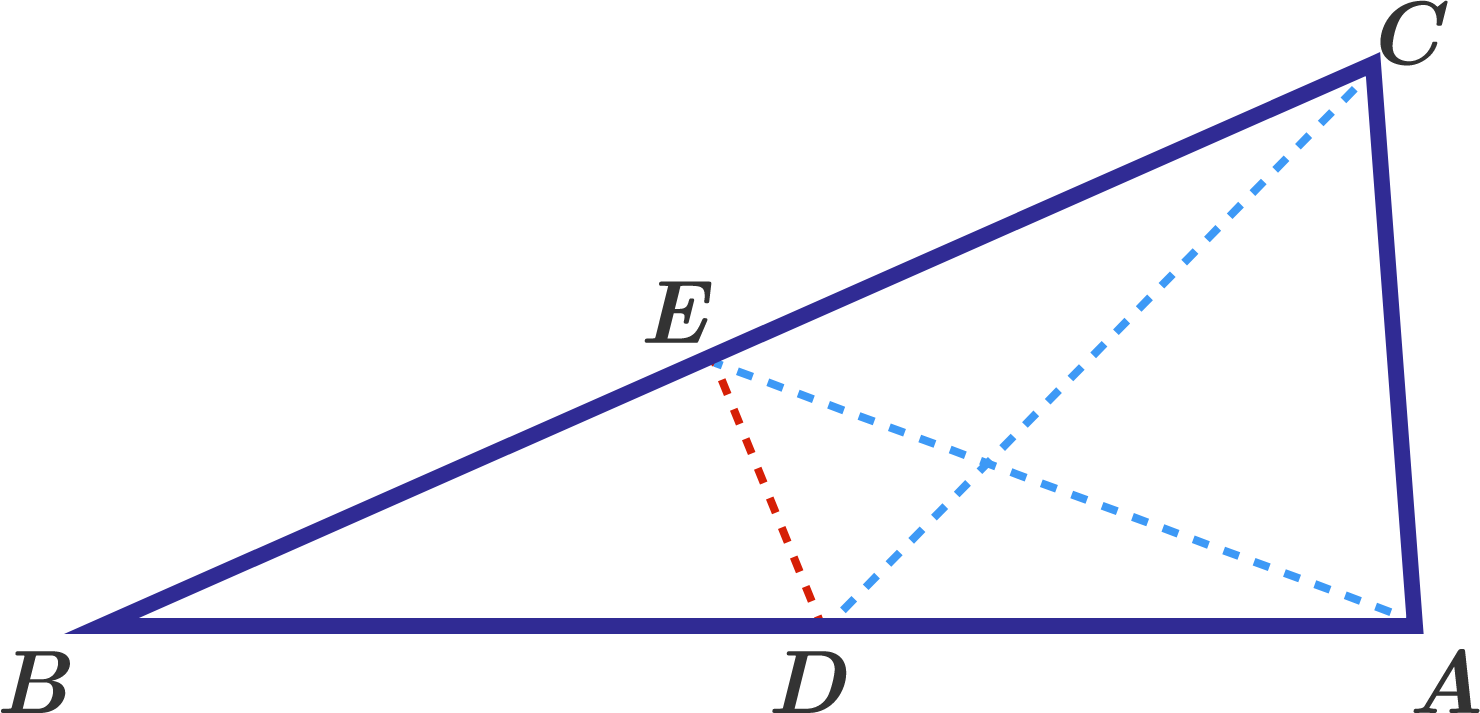In a triangle $$ABC$$ $$\angle A =84^\circ,$$ $$\angle C=78^\circ.$$ Points $$D$$ and $$E$$ are taken on the sides $$AB$$ and $$BC,$$ so that $$\angle ACD =48^\circ,$$ $$\angle CAE =63^\circ.$$ What is the measure (in degrees) of $$\angle CDE$$ ?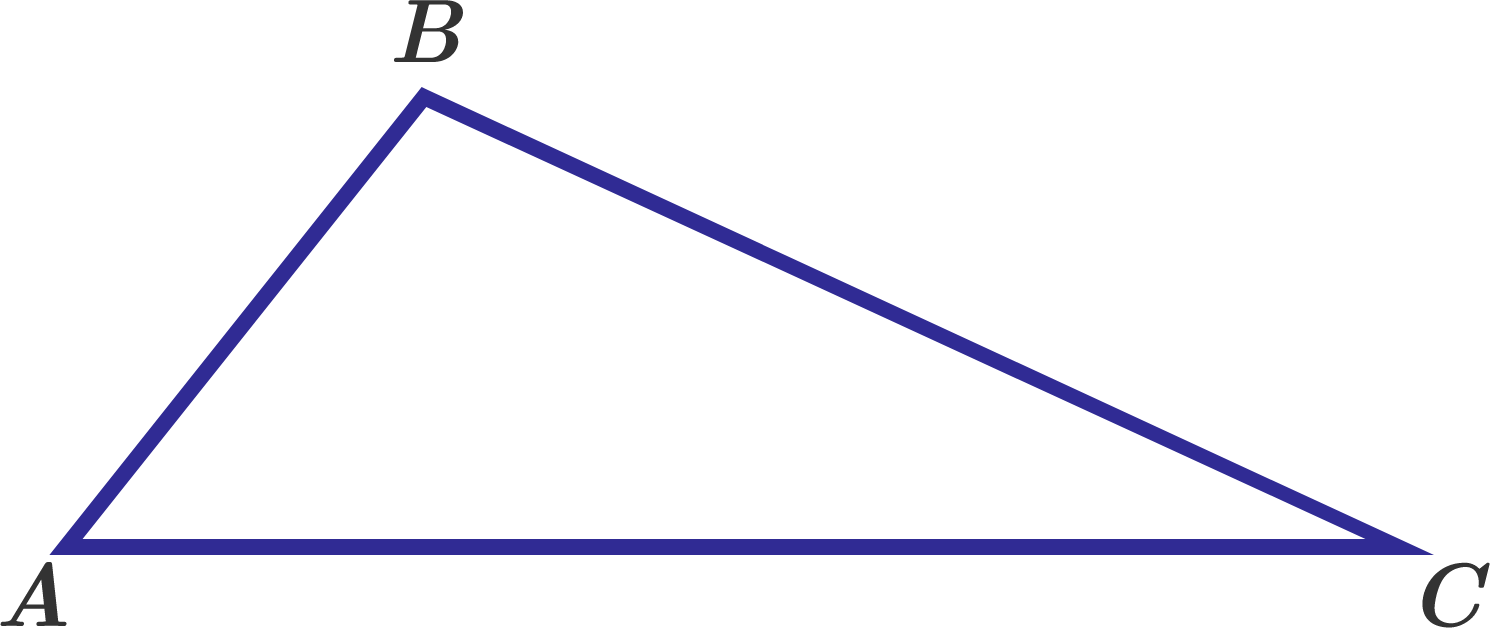In triangle $$ABC$$, $$\cos A : \cos B : \cos C = 2 : 9 : 12$$.

If $$\sin A : \sin B : \sin C = p : q : r$$, where $$p$$, $$q$$ and $$r$$ are coprime positive integers, find $$pqr-(p+q+r)$$.

Triangle $$ABC$$ has area equal to $$\dfrac {90 \sqrt{3}}{4}$$ and perimeter equal to $$30.$$ Also, one of its angles is equal to $$60^\circ.$$ What is the product of the sides of $$ABC?$$

×